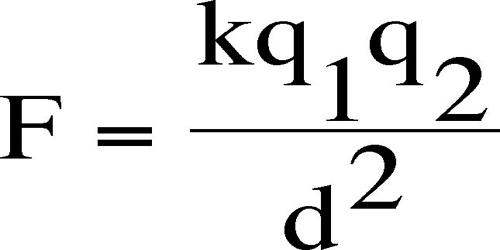# Explain Coulomb’s Inverse Square Law

Coulomb’s inverse square law states that the force of attraction or repulsion between the two magnetic poles is directly proportional to the product of their pole strengths and inversely proportional to the square of the distance between them. It means that if there are two charged bodies one is positively charged and one is negatively charged, then they will attract each other if they are kept at a certain distance from each other.

Now if we increase the charge of one body keeping other unchanged, the attraction force is obviously increased. Similarly, if we increase the charge of the second body keeping the first one unchanged, the attraction force between them is again increased. Hence, the force between the charged bodies is proportional to the charge of either body or both. Columb’s law states that force of interaction is directly proportional to the product of two charges and inversely proportional to the square of the distance between them.

F = K m1m2/ d2.

Where, K = constant it is equal to 9 x 109

If m1 and m2 are the pole strengths of two magnetic poles separated by a distance of d in a medium, then

F ∞ m1m2 and F ∞ 1/d2

so, F ∞ m1m2/d2

F = k (m1m2/d2)

where k is the constant of proportionality and K = μ/4π, where μ is the permeability of the medium.

but, μ = μ0 * μr

so, μr = μ / μ0

where μr = relative permeability of the medium.

μ0 = permeability of free space or vacuum.

let, m1 = m2 = 1; and d = 1m

we know, K = μ/4π

In free space, μ0 = 4π * 10-7 Hm-1

then, F = (10-7 * m1 * m2)/d2

so, F = 10-7 N (let, m1 = m2 = 1; and d = 1m)

Therefore, the unit pole is defined as that pole which when placed at a distance of 1 meter in free space or air from an equal and similar pole, repels it with a force of 10-1 N.

Here, the magnitude of the electrostatic force of attraction or repulsion between two point charges is directly proportional to the product of the magnitudes of charges and inversely proportional to the square of the distance between them. The force is along the straight line joining them.

There are three requirements which must be met in order to use Coulomb’s law:

• The charges must be stationary with respect to each other.
• The charges must be non-overlapping.
• The charges must be either point charges or else otherwise spherically symmetrical in shape.